# Tendon Profile

## Function

• Define a tendon profile along the length of elements. Create the tendon profile relative to its own coordinate system, which will then be inserted relative to the elements.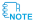Tendon placement can be simplified by copying, rotating and sloping tendon profiles.The modification of the Tendon Profile can be done by directly editing the properties at the Tendon Profile location in the Works Tree.

## Call

From main menu, select [Load] tab > [Type : Temp./Prestress] > [Prestress Loads] group >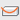[Tendon Profile]

## Input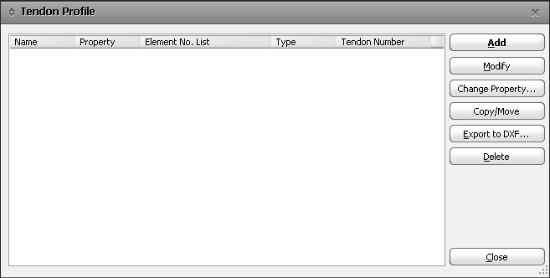If a Tendon Profile is selected from the Tendon Profile List, the tendon placement is displayed on the screen.

Input a new arrangement for the tendons.

### Modify

Modify the defined arrangement of tendons.

### Change Property

Tendon properties assigned to a number of Tendon Profiles can be changed at the same time.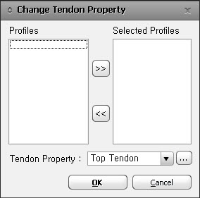### Copy/Move

Copy or Move the inputted Tendon Profile.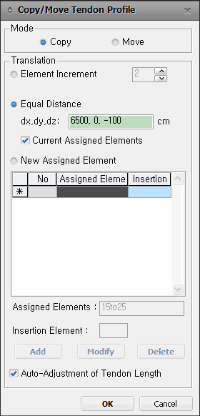### Export to DXF...

Tendon Profile can be exported to DXF as a 2D format (x-z Plane and x-y Plane).If there are a number of Profiles, which are required to have Tendon Properties changed, this functionality allows us to change them simultaneously, rather than changing them one by one in each profile.

### Mode

Copy : Copy the selected Tendon Profile

Move : Copy the selected Tendon Profile

### Translation

Select one of the following options to Copy/Move the selected Tendon Profile

Element Increment : Copy/Move the selected Tendon Profile as per the inputted increment of element number. To Copy/Move the tendon profile defined with this option, at 1, 3, 5, 7 (G1), the following conditions should be satisfied.

The increment of each corresponding element should be identical
For example, Tendon Profile entered in element 1, 3, 5, 7 (G1) can be Copied/Moved to element 51, 53, 55, 57 (G2) because the increment is identical. On the other hand, the Tendon Profile cannot be Copied/Moved to 11, 12, 13, 14 (G3) because the increment is different for each element.

The number of elements to Copy/Move should be the same
For example, the Tendon Profile entered in element 1, 3, 5, 7 (G1) can be Copied/Moved to G4 which has the same number of elements. On the other hand, Tendon Profile cannot be Copied/Moved to G5 since the number of elements is different.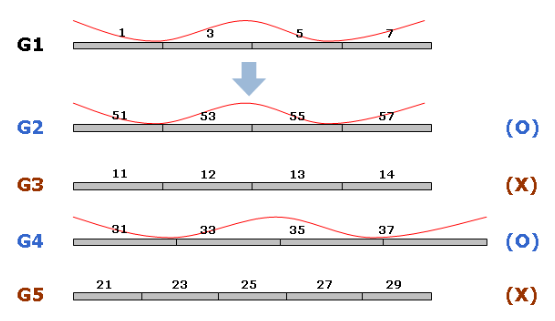Element Increment Option1. Element Increment option can be selected only when the Tendon Profile is defined.

2. Element Increment option is a change of element that is included in Assigned Elements and Insertion Point.

Equal Distance : Enter the absolute distance to Copy/Move the Tendon profile to a particular location. While using this option, the following conditions should be satisfied.

At the distance inputted to Copy/Move the Tendon Profile from the initial Insertion Point of the original element, a node of the element where the Tendon Profile is to be Copied/Moved must exist. This node will define the new Insertion Point. If this node does not exist, then an error message will be displayed.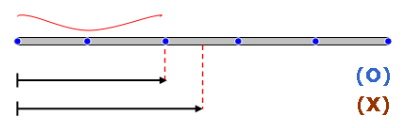If there is more than one element attached to the initial element, then the Tendon Profile will be assigned to the attached element that is most linear.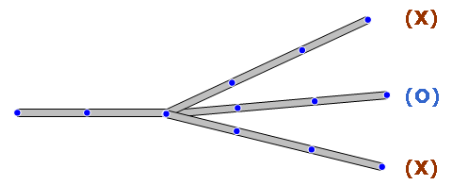If Straight/Curve type Tendon Profile is to be Copied/Moved, then the original length and number of elements should be equal to the length and number of elements at the new location.

Current Assigned Element : It is used to copy Tendon Profile within an element. This option is useful when generating Web Tendons which exist at different locations of the same element. With this option off, tendons only can be copied if another element exists at the distance specified. However, with this option on, it is possible to copy Tendon Profile irrespective of element existence.

New Assigned Element : Input new Assigned Elements and Insertion Point to Copy/Move a Tendon Profile to those elements.

If a Tendon Profile is Copied/Moved to an element whose total length is different from the total length of the original element, then checking this option will automatically adjust the tendon length based on the ratio of the original element length to the length of the element where the Tendon Profile is copied.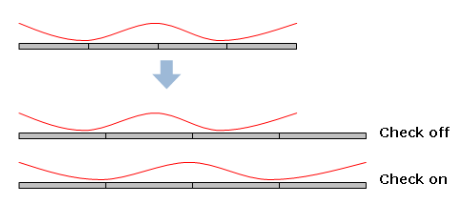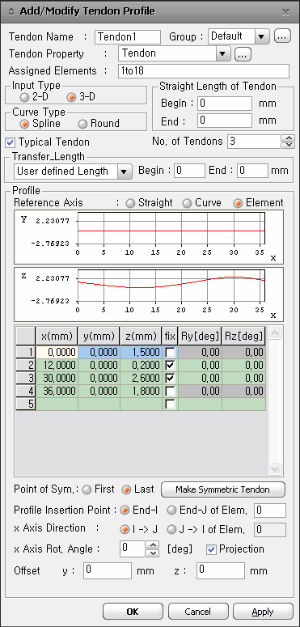Tendon Profile definition dialog box

### Tendon Name

Tendon nameName assigned within 20 alphanumeric characters.

### Tendon Property

Define the tendon properties. Click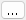to the right to add new, modify or delete previously defined tendon properties.

### Assigned Elements

Specify the element numbers to which the tendon will be assigned. Use the Graphical Selection function of midas Civil to automatically specify the numbers by selecting the elements on the screen.

### Input Type

The dimension of Tendon Profile is specified.

2-D : Tendon Profile is defined in 2-dimensional coordinates.

3-D : Tendon Profile is defined in 3-dimensional coordinates.

### Curve Type

Specify the type of curvature for tendon placement.

Spline : Calculate the minimum polynomial curvature connecting the points defining the tendon profile and auto-place the tendons.

Round : Place the tendons following the circle, which forms tangents to the straight lines connecting the points defining the tendon profile.For External Tendons, they are arranged in a straight line. You can check the arrangement of tendons by enabling the "Tendon Profile" option in the "Misc" tab of the Display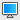settings.

### Straight Length of Tendon

Specify the straight portions of the tendon at both ends.

Begin : At the beginning of the tendon

End : At the end of the tendon

### Typical Tendon

This is a function for defining a lumped representative tendon. If this option is checked on and the number of tendons is inputted, the generated tendon profile is multiplied by the inputted number of tendons when analysis is performed. For simple analysis, such as the schematic design of a bridge, inputting 3 dimensional tendon profiles is time consuming. Therefore, using the function of lumped representative tendon, which defines only one tendon profile for analysis, the time required for generating the model and performing analysis can be reduced.

After analysis is performed, the tendon results, like tendon loss, elongation etc. are displayed as one tendon.

No. of Tendon : Number of lumped representative tendons

### Transfer Length

Enter a Transfer Length. In both pre tensioned and post-tensioned beams, compressive stress due to tendon prestressing forces is not fully distributed to the top and bottom fibers of the member near the end anchorage zones or beam ends over a Transfer Length.

Enter a Transfer Length. In both pre tensioned and post-tensioned beams, compressive stress due to tendon prestressing forces is not fully distributed to the top and bottom fibers of the member near the end anchorage zones or beam ends over a Transfer Length.

User Defined Length : Enter a Transfer Length manually.

Begin : Beginning portion of the Transfer Length

End : End portion of the Transfer Length

Auto Calc(0.5x(H+Bf/n) : For Post-tensioning, the transfer length is auto-calculated as below.

L=0.5x(H+Bf/n)

where, H: Section depth, Bf: Flange width, n: Number of webs (2 for 1Cell)

### Profile

Place the tendon profile by defining the coordinates of the tendon. The tendon coordinate system (TCS) used here is temporary, and its origin is the starting point of the tendon, which will be related to the elements by the profile insertion point. As many coordinates as required to define the profile may be specified, but at least two (start and end) points are required. The x-axis of TCS is parallel with a Global axis in the length direction, and the z-axis of TCS coincides with the Global Z-axis. See Tendon Shape below for further details.

Reference Axis

You can choose the method for inputting tendon coordinates.

Straight : The x-direction (the reference line from which tendon coordinates are defined) of TCS for tendon placement is considered as a straight line.

Curved : The x-direction (the reference line from which tendon coordinates are defined) of TCS for tendon placement is considered as a curved line.

Element : Tendon location is converted into Element Coordinate System and applied.If you define the Tendon Profile based on the Element Type, the tendon length is calculated with respect to the element length. In cases where there is significant curvature, the calculated strain values may be inaccurate. It is recommended to define the Tendon Profile using either the Straight or Curve Type when dealing with highly curved tendons.When the Tendon Profile is defined based on the Element Type, the losses due to tendon curvature friction (curvature friction) are calculated along the axis of the element. Only the curvature in the Local-y and Local-z directions relative to the Local-x axis of the element is considered, and the effect of bridge alignment changes on curvature friction cannot be reflected. In other words, the curvature friction is calculated as if the curved alignment is flattened into a straight line.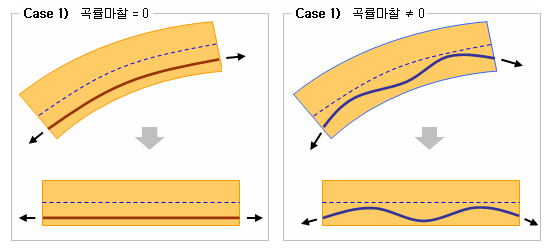The virtual coordinate system (Tendon Coordinate System) is defined such that its x-axis is parallel to an arbitrary axis in the global coordinate system and aligned with the length direction of the tendon. The z-axis is parallel to the global z-axis.

If Spline is selected in Curve Type

If Spline is selected in Curve Type : The tendon is placed along a curve that passes through the user-defined points while ensuring that the curvature is minimized.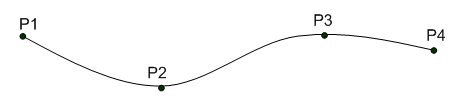1. If the Input Type is set to 3D, you input the coordinates of the tendon points in 3D space along with the radius at each point.

x, y, z : Tendon coordinates in TCS

fix : Check in the box to specify the tangent to the tendon curvature at the point in question.

Ry : If fix is checked, the angle of the tangent line relative to the x-axis in the TCS x-z Plane

Rz : If fix is checked, the angle of the tangent line relative to the x-axis in the TCS x-y Plane

2. If the Input Type is set to 2D, you input the coordinates of the tendon points in 2D space along with the radius at each point.

x, y : The coordinates of the points where the tendon passes through in the x-y plane of the tendon coordinate system.

x, z : The coordinates of the points where the tendon passes through in the x-z plane of the tendon coordinate system.

fix : The tangent angle of the tendon at the respective locations is fixed.

Ry : The tangent angle with the x-axis in the x-z plane of the tendon coordinate system is specified when "fix" is checked.

Rz : The tangent angle with the x-axis in the x-y plane of the tendon coordinate system is specified when "fix" is checked.

BOT : When "BOT" is checked, the distance between the checked points is maintained in terms of the z-coordinate and the distance from the bottom of the section. If there is a difference in distance from the bottom of the section between the two points checked by "BOT," it is interpolated linearly.

However, it is not possible to check in the following cases:

In case of Spline : It is not possible to check BOT if the point is included in a straight segment (as specified in the Straight Length of Tendon input).

In case of Round : It is not possible to check BOT if the point has a specified value for R.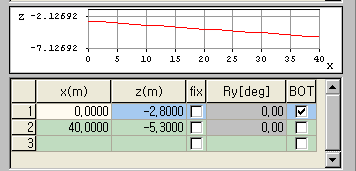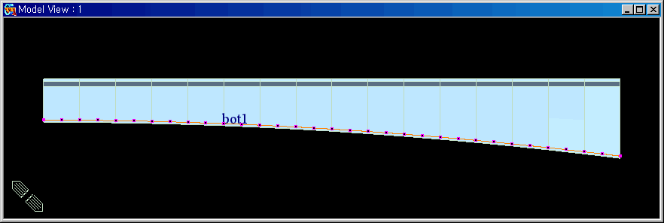The Tendon Profile following the bottom of the section is defined using the BOT option.

If Round is selected in Curve Type

If Round is selected in Curve Type : When Round of Curve Type is selected, the tendon profile is created using straight lines and arcs.1. For Input Type 3D, the Tendon Profile is defined in 3-dimensional space. The coordinates of Tendon Points and their respective radii are inputted for each point.

x,y,z : Input the coordinates of points through which the tendon passes.

R : Enter the radius of an arc at the corresponding point.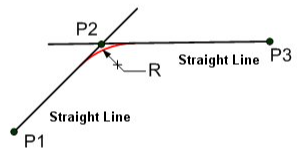2. In the case of 2D Input Type, the Tendon Profile is defined in the x-y plane and the x-z plane separately.

x,y,z : Input the coordinates of points through which the tendon passes.

R : The radius of the arc at the given point.

Using this option, you can create the following tendon shape by inputting only two points, P1 and P2.

Right : Generate a point to the right of the entered point.

Left : Generate a point to the left of the entered point.

A[deg] : Angle formed from the line connecting the entered point and the additionally created point to the x-axis. The upward direction is (+) and the downward direction is (-) with reference to the x-axis.

h : Distance from the entered point to the additionally created point in the y or z-direction. (Distance should be a positive value.)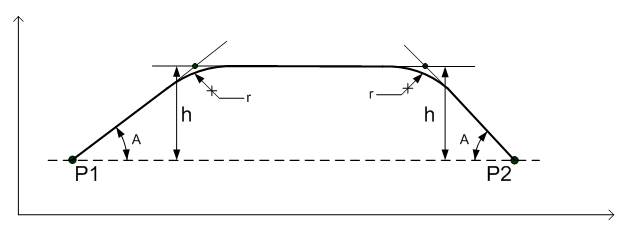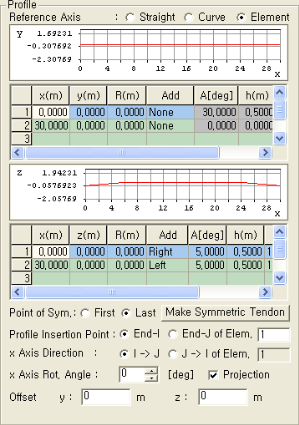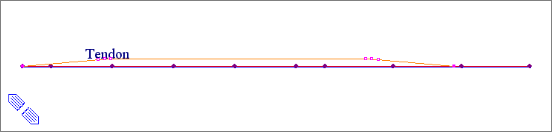Example

After inputting the coordinates of the two end points, create additional points by selecting "Right" for the "Add" option at Point 1 and "Left" for Point 2. Then, input the angle, distance, radius, and other parameters for each point. By doing so, you can obtain the following tendon profile shape.

Point of Sym.

First : The starting point of the current Tendon is assumed to be the axis of symmetry.

Last : The end point of the current Tendon is assumed to be the axis of symmetry.

Make Symmetric Tendon : Tendon Profile is created on the basis of the selected symmetry point (First or Last).

If Straight is selected in Reference Axis

If Straight is selected in Reference Axis

Profile Insertion Point

The origin of the tendon coordinate system (start point of tendon) is identified and inserted into the actual location in the global coordinate system.

x Axis Direction

In case the Reference Axis is Straight, define the x-axis of TCS for tendon placement.

X : x-axis of TCS is parallel with GCS X-axis

Y : x-axis of TCS is parallel with GCS Y-axis

Vector : Define the TCS x-direction by a Vector specified in the entry field below  .

x Axis Rot. Angle

The tendon profile placed in the table is rotated about TCS x-axis by the Angle. This is usefully implemented when placing a tendon on an inclined (non-vertical) web.

Projection

The tendon profile placed in the table is projected on the plane, which is rotated about TCS x-axis by the Angle.

The tendon placement is rotated about the GCS Y or X-axis. This is a useful feature when attempting to place the tendon in a section, which is tilted (rotated) about its local x-axis.Tendons can be placed only in beam elements. The assigned beam elements do not necessarily have to be interconnected.

If Curve is selected in Reference Axis

If Curve is selected in Reference Axis

Profile Insertion Point

The origin of the tendon coordinate system (start point of tendon) is identified and inserted into the actual location in the global coordinate system.

In case the reference axis is a Curve, enter the coordinates of the center of the circle in GCS.

Offset : Place the tendon at the projected location in the radial directionIn the case of a Curved Tendon Shape, the tendon is placed along the circumference of the circle defined by the radius formed by the start point of the tendon (origin of TCS) and the center of the circle. The Offset increases or decreases the radius by the specified magnitude while maintaining the center of the circle. Again the tendon is placed along the changed circumference. When several tendons are placed side by side, use the Copy function to copy the previously entered tendon and relocate the copied tendon by Offset.

Direction

CW : Define the curve clockwise

CCW : Define the curve counter-clockwise

x Axis Rot. Angle

The tendon profile placed in the table is rotated about TCS x-axis by the Angle. This is usefully implemented when placing a tendon on an inclined (non-vertical) web.x Axis Rot. Angle must be entered between -85 and +85 degrees.

Projection

The tendon profile placed in the table is projected on the plane, which is rotated about TCS x-axis by the Angle.

The tendon placement is rotated about the GCS Y or X-axis. This is a useful feature when attempting to place the tendon in a section, which is tilted (rotated) about its local x-axis.Tendons can be placed only in beam elements. The assigned beam elements do not necessarily have to be interconnected.

If Element is selected in Reference Axis

If Element is selected in Reference Axis

Profile Insertion Point

Specify the corresponding element number at which the tendon starts.

End-I of Elem.: I-end of the specified Element is referenced as the insertion point.

End-J of Elem.: J-end of the specified Element is referenced as the insertion point.

x Axis Direction

In case the Reference Axis is an Element, define the x-axis of TCS (Tendon Coordinate System) for tendon placement.

I->J of Elem. : The x-axis in TCS is defined in the direction from the I-end to J-end of the element.

J->I of Elem. : The x-axis in TCS is defined in the direction from the J-end to I-end of the element.

x Axis Rot. Angle

The tendon profile placed in the table is rotated about TCS x-axis by the Angle. This is usefully implemented when placing a tendon on an inclined (non-vertical) web.

Projection

The tendon profile placed in the table is projected on the plane, which is rotated about TCS x-axis by the Angle. The projection direction is in the TCS y-axis prior to rotation.

Offset : Tendon Offset is defined in the Element Coordinate System (ECS).

y : Distance by which the tendon profile is moved in the ECS y-axis direction

z : Distance by which the tendon profile is moved in the ECS z-axis direction

0